# CI Controller

Compression-ignition controller that includes air mass flow, torque, and EGR estimation

• Library:
• Powertrain Blockset / Propulsion / Combustion Engine Controllers

•## Description

The CI Controller block implements a compression-ignition (CI) controller with air mass flow, torque, exhaust gas recirculation (EGR) flow, exhaust back-pressure, and exhaust gas temperature estimation. You can use the CI Controller block in engine control design or performance, fuel economy, and emission tradeoff studies. The core engine block requires the commands that are output from the CI Controller block.

The block uses the commanded torque and measured engine speed to determine these open-loop actuator commands:

• Injector pulse-width

• Fuel injection timing

• Variable geometry turbocharger (VGT) rack position

• EGR valve area percent

The CI Controller block has two subsystems:

• The `Controller` subsystem — Determines the commands based on tables that are functions of commanded torque and measured engine speed.

Based OnDetermines Commands for

Commanded torque

Measured engine speed

Injector pulse-width

Fuel injection timing

VGT rack position

EGR valve area percent

• The `Estimator` subsystem — Determines estimates based on these engine attributes.

Based OnEstimates

Measured engine speed

Fuel injection timing

Cycle average intake manifold pressure and temperature

Fuel injector pulse-width

Absolute ambient pressure

EGR valve area percent

VGT rack position

VGT speed

Air mass flow

Torque

Exhaust gas temperature

Exhaust gas back-pressure

EGR valve gas mass flow

The figure illustrates the signal flow.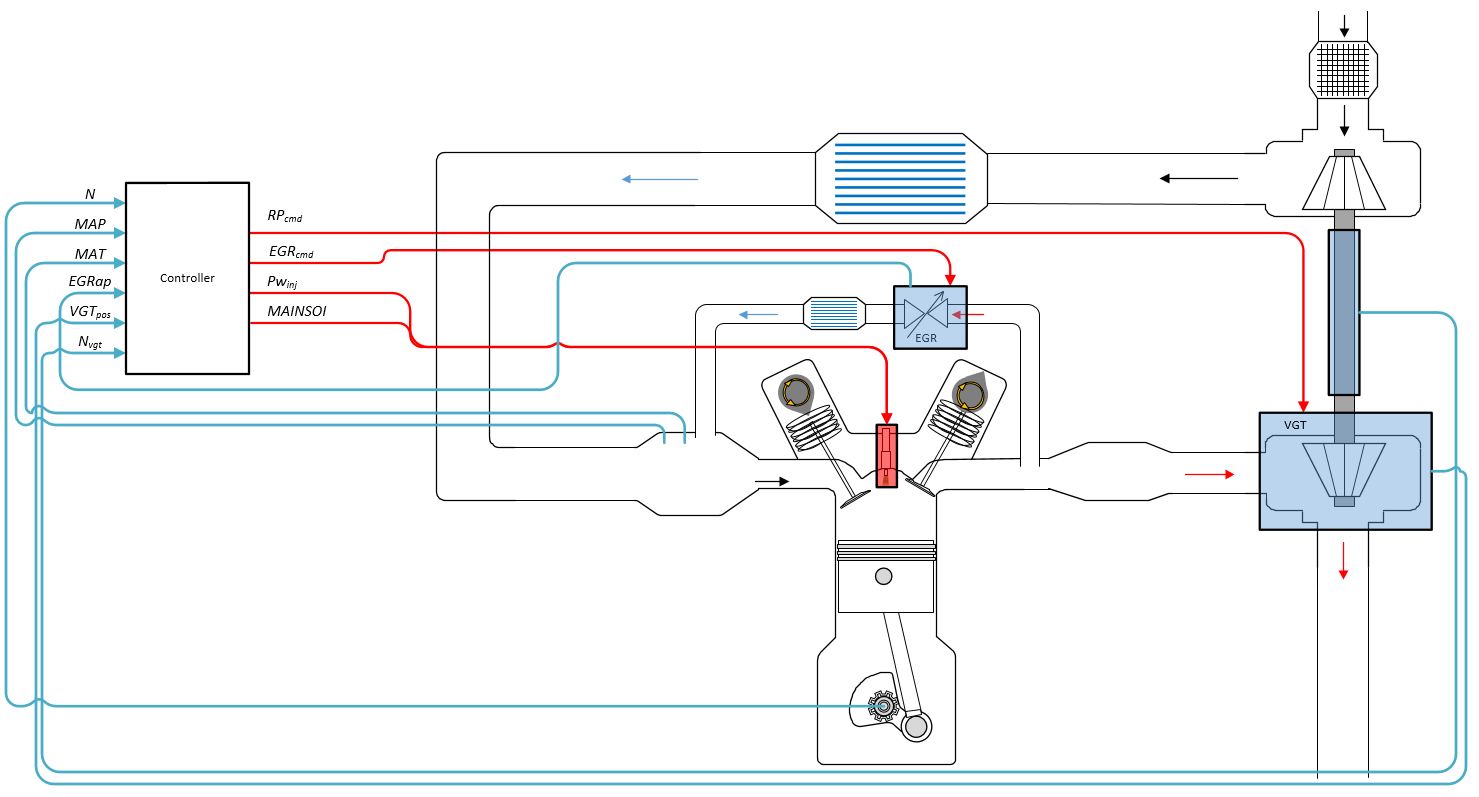The figure uses these variables.

 N Engine speed MAP Cycle average intake manifold absolute pressure MAT Cycle average intake manifold gas absolute temperature EGRap, EGRcmd EGR valve area percent and EGR valve area percent command, respectively VGTpos VGT rack position Nvgt Corrected turbocharger speed RPcmd VGT rack position command $P{w}_{inj}$ Fuel injector pulse-width MAINSOI Start of injection timing for main fuel injection pulse

The Model-Based Calibration Toolbox™ was used to develop the tables that are available with the Powertrain Blockset™.

### Controller

The controller governs the combustion process by commanding VGT rack position, EGR valve area percent, fuel injection timing, and injector pulse-width. Feedforward lookup tables, which are functions of measured engine speed and commanded torque, determine the control commands.

Air

The controller commands the EGR valve area percent and VGT rack position. Changing the VGT rack position modifies the turbine flow characteristics. At low-requested torques, the rack position can reduce the exhaust back pressure, resulting in a low turbocharger speed and boost pressure. When the commanded fuel requires additional air mass flow, the rack position is set to close the turbocharger vanes, increasing the turbocharger speed and intake manifold boost pressure.

The variable geometry turbocharger (VGT) rack position lookup table is a function of commanded torque and engine speed

`$R{P}_{cmd}={f}_{RPcmd}\left(Tr{q}_{cmd},N\right)$`

where:

• RPcmd is VGT rack position command, in percent.

• Trqcmd is commanded engine torque, in N·m.

• N is engine speed, in rpm.The commanded exhaust gas recirculation (EGR) valve area percent lookup table is a function of commanded torque and engine speed

`$EG{R}_{cmd}={f}_{EGRcmd}\left(Tr{q}_{cmd},N\right)$`

where:

• EGRcmd is commanded EGR valve area percent, in percent.

• Trqcmd is commanded engine torque, in N·m.

• N is engine speed, in rpm.Fuel

To initiate combustion, a CI engine injects fuel directly into the combustion chamber. After the injection, the fuel spontaneously ignites, increasing cylinder pressure. The total mass of the injected fuel and main injection timing determines the torque production.

Assuming constant fuel rail pressure, the CI controller commands the injector pulse-width based on the total requested fuel mass:

The equation uses these variables.

 $P{w}_{inj}$ Fuel injector pulse-width Sinj Fuel injector slope Fcmd,tot Commanded total fuel mass per injection MAINSOI Main start-of-injection timing N Engine speed

The commanded total fuel mass per injection table is a function of the torque command and engine speed

`${F}_{cmd,tot}={f}_{Fcmd,tot}\left(Tr{q}_{cmd},N\right)$`

where:

• Fcmd,tot = F is commanded total fuel mass per injection, in mg per cylinder.

• Trqcmd is commanded engine torque, in N·m.

• N is engine speed, in rpm.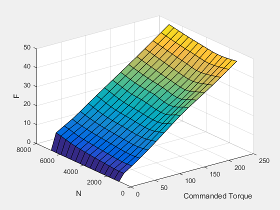The main start-of-injection (SOI) timing lookup table is a function of commanded fuel mass and engine speed

`$MAINSOI=f\left({F}_{cmd,tot},N\right)$`

where:

• MAINSOI is the main start-of-injection timing, in degrees crank angle after top dead center (degATDC).

• Fcmd,tot = F is commanded fuel mass, in mg per injection.

• N is engine speed, in rpm.Idle Speed

When the commanded torque is below a threshold value, the idle speed controller regulates the engine speed.

IfIdle Speed Controller
Trqcmd,input < Trqidlecmd,enableEnabled
Trqidlecmd,enableTrqcmd,inputNot enabled

The idle speed controller uses a discrete PI controller to regulate the target idle speed by commanding a torque.

The PI controller uses this transfer function:

`${C}_{idle}\left(z\right)={K}_{p,idle}+{K}_{i,idle}\frac{{t}_{s}}{z-1}$`

The idle speed commanded torque must be less than the maximum commanded torque:

0 ≤ TrqidlecomdTrqidlecmd,max

Idle speed control is active under these conditions. If the commanded input torque drops below the threshold for enabling the idle speed controller (Trqcmd,input < Trqidlecmd,enable), the commanded engine torque is given by:

Trqcmd = max(Trqcmd,input,Trqidlecmd).

The equations use these variables.

 Trqcmd Commanded engine torque Trqcmd,input Input commanded engine torque Trqidlecmd,enable Threshold for enabling idle speed controller Trqidlecmd Idle speed controller commanded torque Trqidlecmd,max Maximum commanded torque Nidle Base idle speed Kp,idle Idle speed controller proportional gain Ki,idle Idle speed controller integral gain

Speed Limiter

To prevent over revving the engine, the block implements an engine speed limit controller that limits the engine speed to the value specified by the Rev-limiter speed threshold parameter on the Controls > Idle Speed tab.

If the engine speed, N, exceeds the engine speed limit, Nlim, the block sets the commanded engine torque to 0.

To smoothly transition the torque command to 0 as the engine speed approaches the speed limit, the block implements a lookup table multiplier. The lookup table multiplies the torque command by a value that ranges from 0 (engine speed exceeds limit) to 1 (engine speed does not exceed the limit).

### Estimator

Using the CI Core Engine block, the CI Controller block estimates the air mass flow rate, EGR valve mass flow, exhaust back-pressure, engine torque, AFR, and exhaust temperature from sensor feedback. The `Info` port provides the estimated values, but block does not use them to determine the open-loop engine actuator commands.

Air Mass Flow

To calculate the air mass flow, the compression-ignition (CI) engine uses the CI Engine Speed-Density Air Mass Flow Model. The speed-density model uses the speed-density equation to calculate the engine air mass flow, relating the engine intake port mass flow to the intake manifold pressure, intake manifold temperature, and engine speed.

EGR Valve Mass Flow

To calculate the estimated exhaust gas recirculation (EGR) valve mass flow, the block calculates the EGR flow that would occur at standard temperature and pressure conditions, and then corrects the flow to actual temperature and pressure conditions. The block EGR calculation uses estimated exhaust back-pressure, estimated exhaust temperature, standard temperature, and standard pressure.

`${\stackrel{˙}{m}}_{egr,est}={\stackrel{˙}{m}}_{egr,std}\frac{{P}_{exh,est}}{{P}_{std}}\sqrt{\frac{{T}_{std}}{{T}_{exh,est}}}$`

• The standard exhaust gas recirculation (EGR) mass flow is a lookup table that is a function of the standard flow pressure ratio and EGR valve flow area

`${\stackrel{˙}{m}}_{egr,std}=f\left(\frac{MAP}{{P}_{exh,est}},EGRap\right)$`

where:

• ${\stackrel{˙}{m}}_{egr,std}$ is the standard EGR valve mass flow, in g/s.

• Pexh,est is the estimated exhaust back-pressure, in Pa.

• MAP is the cycle average intake manifold absolute pressure, in Pa.

• EGRap is the measured EGR valve area, in percent.The equations use these variables.

 ${\stackrel{˙}{m}}_{egr,est}$ Estimated EGR valve mass flow ${\stackrel{˙}{m}}_{egr,std}$ Standard EGR valve mass flow ${P}_{std}$ Standard pressure ${T}_{std}$ Standard temperature Texh,est Estimated exhaust manifold gas temperature MAP Measured cycle average intake manifold absolute pressure Pexh,est Estimated exhaust back-pressure ${P}_{Amb}$ Absolute ambient pressure EGRap Measured EGR valve area percent

Exhaust Back-Pressure

To estimate the EGR valve mass flow, the block requires an estimate of the exhaust back-pressure. To estimate the exhaust back-pressure, the block uses the ambient pressure and the turbocharger pressure ratio.

`${P}_{exh,est}={P}_{Amb}P{r}_{turbo}$`

For the turbocharger pressure ration calculation, the block uses two lookup tables. The first lookup table determines the approximate turbocharger pressure ratio as a function of turbocharger mass flow and corrected turbocharger speed. Using a second lookup table, the block corrects the approximate turbocharger pressure ratio for VGT rack position.

`$\begin{array}{l}P{r}_{turbo}=f\left({\stackrel{˙}{m}}_{airstd},{N}_{vgtcorr}\right)f\left(VG{T}_{pos}\right)\\ \text{where:}\\ {N}_{vgtcorr}=\frac{{N}_{vgt}}{\sqrt{{T}_{exh,est}}}\end{array}$`

The equations use these variables.

 ${\stackrel{˙}{m}}_{egr,est}$ Estimated EGR valve mass flow ${\stackrel{˙}{m}}_{egr,std}$ Standard EGR valve mass flow ${\stackrel{˙}{m}}_{port,est}$ Estimated intake port mass flow rate ${\stackrel{˙}{m}}_{airstd}$ Standard air mass flow EGRap Measured EGR valve area MAP Measured cycle average intake manifold absolute pressure MAT Measured cycle average intake manifold gas absolute temperature ${P}_{std}$ Standard pressure ${T}_{std}$ Standard temperature Texh,est Estimated exhaust manifold gas temperature Prvgtcorr Turbocharger pressure ratio correction for VGT rack position Prturbo Turbocharger pressure ratio Pexh,est Estimated exhaust back-pressure ${P}_{Amb}$ Absolute ambient pressure Nvgtcorr Corrected turbocharger speed VGTpos Measured VGT rack position

The exhaust-back pressure calculation uses these lookup tables:

• The turbocharger pressure ratio, corrected for variable geometry turbocharger (VGT) speed, is a lookup table that is a function of the standard air mass flow and corrected turbocharger speed, $P{r}_{turbo}=f\left({\stackrel{˙}{m}}_{airstd},{N}_{vgtcorr}\right)$, where:

• Prturbo is the turbocharger pressure ratio, corrected for VGT speed.

• ${\stackrel{˙}{m}}_{airstd}$ is the standard air mass flow, in g/s.

• Nvgtcorr is the corrected turbocharger speed, in rpm/K^(1/2).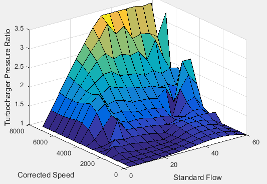To calculate the standard air mass flow through the turbocharger, the block uses conservation of mass, the estimated intake port, and EGR mass flows (from the last estimated calculation). The calculation assumes negligible exhaust manifold filling dynamics.

`${\stackrel{˙}{m}}_{airstd}=\left({\stackrel{˙}{m}}_{port,est}-{\stackrel{˙}{m}}_{egr,est}\right)\frac{{P}_{std}}{MAP}\sqrt{\frac{MAT}{{T}_{std}}}$`

• The variable geometry turbocharger pressure ratio correction is a function of the rack position, Prvgtcorr= ƒ(VGTpos), where:

• Prvgtcorr is the turbocharger pressure ratio correction.

• VGTpos is the variable geometry turbocharger (VGT) rack position.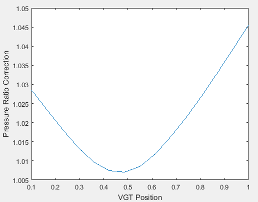Engine Torque

To calculate the engine torque, you can configure the block to use either of these torque models.

Brake Torque ModelDescription
CI Engine Torque Structure Model

The CI core engine torque structure model determines the engine torque by reducing the maximum engine torque potential as these engine conditions vary from nominal:

• Start of injection (SOI) timing

• Exhaust back-pressure

• Burned fuel mass

• Intake manifold gas pressure, temperature, and oxygen percentage

• Fuel rail pressure

To account for the effect of post-inject fuel on torque, the model uses a calibrated torque offset table.

CI Engine Simple Torque Model

For the simple engine torque calculation, the CI engine uses a torque lookup table map that is a function of engine speed and injected fuel mass.

Exhaust Temperature

The exhaust temperature calculation depends on the torque model. For both torque models, the block implements lookup tables.

Torque Model

Description

Equations

`Simple Torque Lookup`

Exhaust temperature lookup table is a function of the injected fuel mass and engine speed.

`${T}_{exh}={f}_{Texh}\left(F,N\right)$`

`Torque Structure`

The nominal exhaust temperature, Texhnom, is a product of these exhaust temperature efficiencies:

• SOI timing

• Intake manifold gas pressure

• Intake manifold gas temperature

• Intake manifold gas oxygen percentage

• Fuel rail pressure

• Optimal temperature

The exhaust temperature, Texhnom, is offset by a post temperature effect, ΔTpost, that accounts for post and late injections during the expansion and exhaust strokes.

`$\begin{array}{l}{T}_{exhnom}=SO{I}_{exhteff}MA{P}_{exhteff}MA{T}_{exhteff}O2{p}_{exhteff}FUEL{P}_{exhteff}Tex{h}_{opt}\\ {T}_{exh}={T}_{exhnom}+\Delta {T}_{post}\\ \\ SO{I}_{exhteff}={f}_{SO{I}_{exhteff}}\left(\Delta SOI,N\right)\\ MA{P}_{exhteff}={f}_{MA{P}_{exhteff}}\left(MA{P}_{ratio},\lambda \right)\\ MA{T}_{exhteff}={f}_{MA{T}_{exhteff}}\left(\Delta MAT,N\right)\\ O2{p}_{exhteff}={f}_{O2{p}_{exhteff}}\left(\Delta O2p,N\right)\\ Tex{h}_{opt}={f}_{Texh}\left(F,N\right)\end{array}$`

The equations use these variables.

 F Compression stroke injected fuel mass N Engine speed Texh Exhaust manifold gas temperature Texhopt Optimal exhaust manifold gas temperature ΔTpost Post injection temperature effect Texhnom Nominal exhaust temperature SOIexhteff Main SOI exhaust temperature efficiency multiplier ΔSOI Main SOI timing relative to optimal timing MAPexheff Intake manifold gas pressure exhaust temperature efficiency multiplier MAPratio Intake manifold gas pressure ratio relative to optimal pressure ratio λ Intake manifold gas lambda MATexheff Intake manifold gas temperature exhaust temperature efficiency multiplier ΔMAT Intake manifold gas temperature relative to optimal temperature O2Pexheff Intake manifold gas oxygen exhaust temperature efficiency multiplier ΔO2P Intake gas oxygen percent relative to optimal FUELPexheff Fuel rail pressure exhaust temperature efficiency multiplier ΔFUELP Fuel rail pressure relative to optimal

Air-Fuel Ratio

The measured engine speed and fuel injector pulse-width determine the commanded fuel mass flow rate:

`${\stackrel{˙}{m}}_{fuel,cmd}=\frac{N{S}_{inj}P{w}_{inj}{N}_{cyl}}{Cps\left(\frac{60s}{min}\right)\left(\frac{1000mg}{g}\right)}$`

The commanded total fuel mass flow and estimated port mass flow rates determine the estimated AFR:

`$AF{R}_{est}=\frac{{\stackrel{˙}{m}}_{port,est}}{{\stackrel{˙}{m}}_{fuel,cmd}}$`

The equations use these variables.

 $P{w}_{inj}$ Fuel injector pulse-width AFRest Estimated air-fuel ratio ${\stackrel{˙}{m}}_{fuel,cmd}$ Commanded fuel mass flow rate ${S}_{inj}$ Fuel injector slope N Engine speed Ncyl Number of engine cylinders Cps Crankshaft revolutions per power stroke, rev/stroke ${\stackrel{˙}{m}}_{port,est}$ Total estimated engine air mass flow at intake ports

## Ports

### Input

expand all

Commanded engine torque, Trqcmd,input, in N·m.

Measured engine speed, N, in rpm.

Measured intake manifold absolute pressure, MAP, in Pa.

Measured intake manifold absolute temperature, MAT, in K.

Absolute ambient pressure, ${P}_{Amb}$, in Pa.

Measured EGR valve area percent, EGRap, in %.

Measured VGT rack position, VGTpos.

Measured VGT speed, Nvgt, in rpm.

Engine cooling temperature, Tcoolant, in K.

State of the vehicle ignition switch, dimensionless.

#### Dependencies

To create this port, on the Stop-Start tab, select Enable Engine Stop-Start.

Command to enable or disable the stop-start logic, dimensionless.

#### Dependencies

To create this port, on the Stop-Start tab, select Enable Engine Stop-Start. Select External Enable Port.

### Output

expand all

Bus signal containing these block calculations.

SignalDescriptionVariableUnits

`InjPw`

Fuel injector pulse-width

$P{w}_{inj}$

ms

`EgrVlvAreaPctCmd`

EGR valve area percent command

EGRcmd%

`TurbRackPosCmd`

VGT rack position command

RPcmdN/A

`TrqCmd`

Engine torque

Trqcmd

N·m

`FuelMassTotCmd`

Commanded total fuel mass per injection

Fcmd,tot

mg

`FuelMainSoi`

Main start-of-injection timing

MAINSOI

degATDC

`FuelMassFlwCmd`

Commanded fuel mass flow rate

${\stackrel{˙}{m}}_{fuel,cmd}$

kg/s

`EstIntkPortMassFlw`

Estimated port mass flow rate

${\stackrel{˙}{m}}_{port,est}$

kg/s

`EstEngTrq`

Estimated engine torque

Trqest

N·m

`EstExhManGasTemp`

Estimated exhaust manifold gas temperature

Texh,est

K

`EstExhPrs`

Estimated exhaust back-pressure

Pex

Pa

`EstEGRFlow`

`EstEGRFlow`

`EstEGRFlow`

`EstEGRFlow`

`EstAfr`

Estimated air-fuel ratio

AFRest

N/A

`EngRevLimAct`

Flag that indicates if rev-limiter control is active

N/A

N/A

Fuel injector pulse-width, $P{w}_{inj}$, in ms.

Main start-of-injection timing, MAINSOI, in degrees crank angle after top dead center (degATDC).

VGT rack position command, RPcmd.

EGR valve area percent command, EGRcmd.

## Parameters

expand all

### Controls

Air - EGR

The commanded exhaust gas recirculation (EGR) valve area percent lookup table is a function of commanded torque and engine speed

`$EG{R}_{cmd}={f}_{EGRcmd}\left(Tr{q}_{cmd},N\right)$`

where:

• EGRcmd is commanded EGR valve area percent, in percent.

• Trqcmd is commanded engine torque, in N·m.

• N is engine speed, in rpm.Commanded torque breakpoints, in N·m.

Speed breakpoints, in rpm.

Air - VGR

The variable geometry turbocharger (VGT) rack position lookup table is a function of commanded torque and engine speed

`$R{P}_{cmd}={f}_{RPcmd}\left(Tr{q}_{cmd},N\right)$`

where:

• RPcmd is VGT rack position command, in percent.

• Trqcmd is commanded engine torque, in N·m.

• N is engine speed, in rpm.Breakpoints, in N·m.

Breakpoints, in rpm.

Fuel

Fuel injector slope, ${S}_{inj}$, in mg/ms.

Stoichiometric air-fuel ratio, AFRstoich.

Fuel lower heating value, in J/kg.

The commanded total fuel mass per injection table is a function of the torque command and engine speed

`${F}_{cmd,tot}={f}_{Fcmd,tot}\left(Tr{q}_{cmd},N\right)$`

where:

• Fcmd,tot = F is commanded total fuel mass per injection, in mg per cylinder.

• Trqcmd is commanded engine torque, in N·m.

• N is engine speed, in rpm.The main start-of-injection (SOI) timing lookup table is a function of commanded fuel mass and engine speed

`$MAINSOI=f\left({F}_{cmd,tot},N\right)$`

where:

• MAINSOI is the main start-of-injection timing, in degrees crank angle after top dead center (degATDC).

• Fcmd,tot = F is commanded fuel mass, in mg per injection.

• N is engine speed, in rpm.Fuel main injection timing fuel breakpoints, in mg per injection.

Fuel main injection timing speed breakpoints, in rpm.

Commanded torque breakpoints, in N·m.

Speed breakpoints, in rpm.

Idle Speed

Base idle speed, Nidle, in rpm.

Torque to enable the idle speed controller, Trqidlecmd,enable, in N·m.

Maximum idle controller commanded torque, Trqidlecmd,max, in N·m.

Proportional gain for idle speed control, Kp,idle, in N·m/rpm.

Integral gain for idle speed control, Ki,idle, in N·m/(rpm·s).

Engine speed limit, Nlim, in rpm.

If the engine speed, N, exceeds the engine speed limit, Nlim, the block sets the commanded engine torque to 0.

To smoothly transition the torque command to 0 as the engine speed approaches the speed limit, the block implements a lookup table multiplier. The lookup table multiplies the torque command by a value that ranges from 0 (engine speed exceeds limit) to 1 (engine speed does not exceed the limit).

Stop-Start

Select to enable the engine stop-start logic. Selecting this option will activate additional parameters to modify the behavior of the Engine Stop-Start block.

Select to add a port to the engine controller block which enables or disables the stop-start logic.

#### Dependencies

To enable this parameter, on the Stop-Start tab, select Enable Engine Stop-Start.

Engine stop time for the stop-start logic, in s.

#### Dependencies

To enable this parameter, on the Stop-Start tab, select Enable Engine Stop-Start.

Catalyst light off time for the stop-start logic, in s.

#### Dependencies

To enable this parameter, on the Stop-Start tab, select Enable Engine Stop-Start.

Sample time for the stop-start logic, in s.

#### Dependencies

To enable this parameter, on the Stop-Start tab, select Enable Engine Stop-Start.

### Estimation

Air

Number of engine cylinders, ${N}_{cyl}$.

Crankshaft revolutions per power stroke, $Cps$, in rev/stroke.

Displaced volume, ${V}_{d}$, in m^3.

Ideal gas constant, ${R}_{air}$, in J/(kg·K).

Standard air pressure, ${P}_{std}$, in Pa.

Standard air temperature, ${T}_{std}$, in K.

The volumetric efficiency lookup table is a function of the intake manifold absolute pressure at intake valve closing (IVC) and engine speed

`${\eta }_{v}={f}_{{\eta }_{v}}\left(MAP,N\right)$`

where:

• ${\eta }_{v}$ is engine volumetric efficiency, dimensionless.

• MAP is intake manifold absolute pressure, in KPa.

• N is engine speed, in rpm.Intake manifold pressure breakpoints for speed-density volumetric efficiency lookup table, in KPa.

Engine speed breakpoints for speed-density volumetric efficiency lookup table, in rpm.

The standard exhaust gas recirculation (EGR) mass flow is a lookup table that is a function of the standard flow pressure ratio and EGR valve flow area

`${\stackrel{˙}{m}}_{egr,std}=f\left(\frac{MAP}{{P}_{exh,est}},EGRap\right)$`

where:

• ${\stackrel{˙}{m}}_{egr,std}$ is the standard EGR valve mass flow, in g/s.

• Pexh,est is the estimated exhaust back-pressure, in Pa.

• MAP is the cycle average intake manifold absolute pressure, in Pa.

• EGRap is the measured EGR valve area, in percent.EGR valve standard flow pressure ratio breakpoints, dimensionless.

EGR valve standard flow area percent breakpoints, in percent.

The turbocharger pressure ratio, corrected for variable geometry turbocharger (VGT) speed, is a lookup table that is a function of the standard air mass flow and corrected turbocharger speed, $P{r}_{turbo}=f\left({\stackrel{˙}{m}}_{airstd},{N}_{vgtcorr}\right)$, where:

• Prturbo is the turbocharger pressure ratio, corrected for VGT speed.

• ${\stackrel{˙}{m}}_{airstd}$ is the standard air mass flow, in g/s.

• Nvgtcorr is the corrected turbocharger speed, in rpm/K^(1/2).Turbocharger pressure ratio standard flow breakpoints, in g/s.

Turbocharger pressure ratio corrected speed breakpoints, in rpm/K^(1/2).

The variable geometry turbocharger pressure ratio correction is a function of the rack position, Prvgtcorr= ƒ(VGTpos), where:

• Prvgtcorr is the turbocharger pressure ratio correction.

• VGTpos is the variable geometry turbocharger (VGT) rack position.Turbocharger pressure ratio VGT position correction breakpoints, dimensionless.

Torque - Simple Torque Lookup

For the simple torque lookup table model, the CI engine uses a lookup table is a function of engine speed and injected fuel mass, ${T}_{brake}={f}_{Tnf}\left(F,N\right)$, where:

• Tq = Tbrake is engine brake torque after accounting for engine mechanical and pumping friction effects, in N·m.

• F is injected fuel mass, in mg per injection.

• N is engine speed, in rpm.#### Dependencies

To enable this parameter, for Torque model, select `Simple Torque Lookup`.

Torque table fuel mass per injection breakpoints, in mg per injection.

#### Dependencies

To enable this parameter, for Torque model, select `Simple Torque Lookup`.

Engine speed breakpoints, in rpm.

#### Dependencies

To enable this parameter, for Torque model, select `Simple Torque Lookup`.

Torque - Torque Structure

Fuel mass per injection breakpoints, in mg per injection.

#### Dependencies

To enable this parameter, for Torque model, select `Torque Structure`.

Engine speed breakpoints, in rpm.

#### Dependencies

To enable this parameter, for Torque model, select `Torque Structure`.

The optimal main start of injection (SOI) timing lookup table, ƒSOIc, is a function of the engine speed and injected fuel mass, SOIc = ƒSOIc(F,N), where:

• SOIc is optimal SOI timing, in degATDC.

• F is compression stroke injected fuel mass, in mg per injection.

• N is engine speed, in rpm.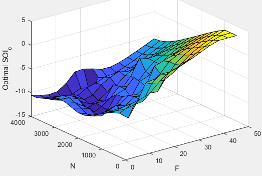#### Dependencies

To enable this parameter, for Torque model, select `Torque Structure`.

The optimal intake manifold gas pressure lookup table, ƒMAP, is a function of the engine speed and injected fuel mass, MAP = ƒMAP(F,N), where:

• MAP is optimal intake manifold gas pressure, in Pa.

• F is compression stroke injected fuel mass, in mg per injection.

• N is engine speed, in rpm.#### Dependencies

To enable this parameter, for Torque model, select `Torque Structure`.

The optimal exhaust manifold gas pressure lookup table, ƒEMAP, is a function of the engine speed and injected fuel mass, EMAP = ƒEMAP(F,N), where:

• EMAP is optimal exhaust manifold gas pressure, in Pa.

• F is compression stroke injected fuel mass, in mg per injection.

• N is engine speed, in rpm.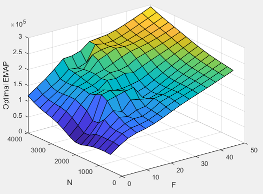#### Dependencies

To enable this parameter, for Torque model, select `Torque Structure`.

The optimal intake manifold gas temperature lookup table, ƒMAT, is a function of the engine speed and injected fuel mass, MAT = ƒMAT(F,N), where:

• MAT is optimal intake manifold gas temperature, in K.

• F is compression stroke injected fuel mass, in mg per injection.

• N is engine speed, in rpm.#### Dependencies

To enable this parameter, for Torque model, select `Torque Structure`.

The optimal intake gas oxygen percent lookup table, ƒO2, is a function of the engine speed and injected fuel mass, O2PCT = ƒO2(F,N), where:

• O2PCT is optimal intake gas oxygen, in percent.

• F is compression stroke injected fuel mass, in mg per injection.

• N is engine speed, in rpm.#### Dependencies

To enable this parameter, for Torque model, select `Torque Structure`.

The optimal fuel rail pressure lookup table, ƒfuelp, is a function of the engine speed and injected fuel mass, FUELP = ƒfuelp(F,N), where:

• FUELP is optimal fuel rail pressure, in MPa.

• F is compression stroke injected fuel mass, in mg per injection.

• N is engine speed, in rpm.#### Dependencies

To enable this parameter, for Torque model, select `Torque Structure`.

The optimal gross indicated mean effective pressure lookup table, ƒimepg, is a function of the engine speed and injected fuel mass, IMEPG = ƒimepg(F,N), where:

• IMEPG is optimal gross indicated mean effective pressure, in Pa.

• F is compression stroke injected fuel mass, in mg per injection.

• N is engine speed, in rpm.#### Dependencies

To enable this parameter, for Torque model, select `Torque Structure`.

The optimal friction mean effective pressure lookup table, ƒfmep, is a function of the engine speed and injected fuel mass, FMEP = ƒfmep(F,N), where:

• FMEP is optimal friction mean effective pressure, in Pa.

• F is compression stroke injected fuel mass, in mg per injection.

• N is engine speed, in rpm.#### Dependencies

To enable this parameter, for Torque model, select `Torque Structure`.

The optimal pumping mean effective pressure lookup table, ƒpmep, is a function of the engine speed and injected fuel mass, PMEP = ƒpmep(F,N), where:

• PMEP is optimal pumping mean effective pressure, in Pa.

• F is compression stroke injected fuel mass, in mg per injection.

• N is engine speed, in rpm.#### Dependencies

To enable this parameter, for Torque model, select `Torque Structure`.

Friction multiplier as a function of temperature, dimensionless.

#### Dependencies

To enable this parameter, for Torque model, select `Torque Structure`.

Friction multiplier temperature breakpoints, in K.

#### Dependencies

To enable this parameter, for Torque model, select `Torque Structure`.

The main start of injection (SOI) timing efficiency multiplier lookup table, ƒSOIeff, is a function of the engine speed and main SOI timing relative to optimal timing, SOIeff = ƒSOIeff(ΔSOI,N), where:

• SOIeff is main SOI timing efficiency multiplier, dimensionless.

• ΔSOI is main SOI timing relative to optimal timing, in degBTDC.

• N is engine speed, in rpm.#### Dependencies

To enable this parameter, for Torque model, select `Torque Structure`.

Main start of injection timing relative to optimal timing breakpoints, in degBTDC.

#### Dependencies

To enable this parameter, for Torque model, select `Torque Structure`.

The intake manifold gas pressure efficiency multiplier lookup table, ƒMAPeff, is a function of the intake manifold gas pressure ratio relative to optimal pressure ratio and lambda, MAPeff = ƒMAPeff(MAPratio,λ), where:

• MAPeff is intake manifold gas pressure efficiency multiplier, dimensionless.

• MAPratio is intake manifold gas pressure ratio relative to optimal pressure ratio, dimensionless.

• λ is intake manifold gas lambda, dimensionless.#### Dependencies

To enable this parameter, for Torque model, select `Torque Structure`.

Intake manifold gas pressure ratio relative to optimal pressure ratio breakpoints, dimensionless.

#### Dependencies

To enable this parameter, for Torque model, select `Torque Structure`.

Intake manifold gas lambda breakpoints, dimensionless.

#### Dependencies

To enable this parameter, for Torque model, select `Torque Structure`.

The intake manifold gas temperature efficiency multiplier lookup table, ƒMATeff, is a function of the engine speed and intake manifold gas temperature relative to optimal temperature, MATeff = ƒMATeff(ΔMAT,N), where:

• MATeff is intake manifold gas temperature efficiency multiplier, dimensionless.

• ΔMAT is intake manifold gas temperature relative to optimal temperature, in K.

• N is engine speed, in rpm.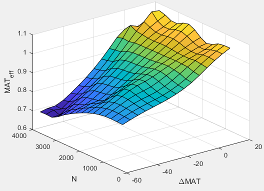#### Dependencies

To enable this parameter, for Torque model, select `Torque Structure`.

Intake manifold gas temperature relative to optimal gas temperature breakpoints, in K.

#### Dependencies

To enable this parameter, for Torque model, select `Torque Structure`.

The intake manifold gas oxygen efficiency multiplier lookup table, ƒO2Peff, is a function of the engine speed and intake manifold gas oxygen percent relative to optimal, O2Peff = ƒO2Peff(ΔO2P,N), where:

• O2Peff is intake manifold gas oxygen efficiency multiplier, dimensionless.

• ΔO2P is intake gas oxygen percent relative to optimal, in percent.

• N is engine speed, in rpm.#### Dependencies

To enable this parameter, for Torque model, select `Torque Structure`.

Intake gas oxygen percent relative to optimal breakpoints, in percent.

#### Dependencies

To enable this parameter, for Torque model, select `Torque Structure`.

The fuel rail pressure efficiency multiplier lookup table, ƒFUELPeff, is a function of the engine speed and fuel rail pressure relative to optimal breakpoints, FUELPeff = ƒFUELPeff(ΔFUELP,N), where:

• FUELPeff is fuel rail pressure efficiency multiplier, dimensionless.

• ΔFUELP is fuel rail pressure relative to optimal, in MPa.

• N is engine speed, in rpm.#### Dependencies

To enable this parameter, for Torque model, select `Torque Structure`.

Fuel rail pressure relative to optimal breakpoints, in MPa.

#### Dependencies

To enable this parameter, for Torque model, select `Torque Structure`.

Fuel mass injection type identifier, dimensionless.

In the CI Core Engine and CI Controller blocks, you can represent multiple injections with the start of injection (SOI) and fuel mass inputs to the model. To specify the type of injection, use the Fuel mass injection type identifier parameter.

Type of InjectionParameter Value

Pilot

`0`

Main

`1`

Post

`2`

Passed

`3`

The model considers `Passed` fuel injections and fuel injected later than a threshold to be unburned fuel. Use the parameter to specify the threshold.

#### Dependencies

To enable this parameter, for Torque model, select `Torque Structure`.

The indicated mean effective pressure post inject correction lookup table, ƒIMEPpost, is a function of the engine speed and fuel rail pressure relative to optimal breakpoints, ΔIMEPpost = ƒIMEPpost(ΔSOIpost,Fpost), where:

• ΔIMEPpost is indicated mean effective pressure post inject correction, in Pa.

• ΔSOIpost is indicated mean effective pressure post inject start of inject timing centroid, in degATDC.

• Fpost is indicated mean effective pressure post inject mass sum, in mg per injection.#### Dependencies

To enable this parameter, for Torque model, select `Torque Structure`.

Indicated mean effective pressure post inject mass sum breakpoints, in mg per injection.

#### Dependencies

To enable this parameter, for Torque model, select `Torque Structure`.

Indicated mean effective pressure post inject start of inject timing centroid breakpoints, in degATDC.

#### Dependencies

To enable this parameter, for Torque model, select `Torque Structure`.

Maximum start of injection angle for burned fuel, in degATDC.

#### Dependencies

To enable this parameter, for Torque model, select `Torque Structure`.

Exhaust

Exhaust gas-specific heat, $C{p}_{exh}$, in J/(kg·K).

Exhaust Temperature - Simple Torque Lookup

The lookup table for the exhaust temperature is a function of injected fuel mass and engine speed

`${T}_{exh}={f}_{Texh}\left(F,N\right)$`

where:

• ${T}_{exh}$ is exhaust temperature, in K.

• F is injected fuel mass, in mg per injection.

• N is engine speed, in rpm.#### Dependencies

To enable this parameter, for Torque model, select `Simple Torque Lookup`.

Engine load breakpoints used for exhaust temperature lookup table, in mg per injection.

#### Dependencies

To enable this parameter, for Torque model, select `Simple Torque Lookup`.

Engine speed breakpoints used for exhaust temperature lookup table, in rpm.

#### Dependencies

To enable this parameter, for Torque model, select `Simple Torque Lookup`.

Exhaust Temperature - Torque Structure

The optimal exhaust manifold gas temperature lookup table, ƒTexh, is a function of the engine speed engine speed and injected fuel mass, Texhopt = ƒTexh(F,N), where:

• Texhopt is optimal exhaust manifold gas temperature, in K.

• F is compression stroke injected fuel mass, in mg per injection.

• N is engine speed, in rpm.#### Dependencies

To enable this parameter, for Torque model, select `Torque Structure`.

The main start of injection (SOI) timing exhaust temperature efficiency multiplier lookup table, ƒSOIexhteff, is a function of the engine speed engine speed and injected fuel mass, SOIexhteff = ƒSOIexhteff(ΔSOI,N), where:

• SOIexhteff is main SOI exhaust temperature efficiency multiplier, dimensionless.

• ΔSOI is main SOI timing relative to optimal timing, in degBTDC.

• N is engine speed, in rpm.#### Dependencies

To enable this parameter, for Torque model, select `Torque Structure`.

The intake manifold gas pressure exhaust temperature efficiency multiplier lookup table, ƒMAPexheff, is a function of the intake manifold gas pressure ratio relative to optimal pressure ratio and lambda, MAPexheff = ƒMAPexheff(MAPratio,λ), where:

• MAPexheff is intake manifold gas pressure exhaust temperature efficiency multiplier, dimensionless.

• MAPratio is intake manifold gas pressure ratio relative to optimal pressure ratio, dimensionless.

• λ is intake manifold gas lambda, dimensionless.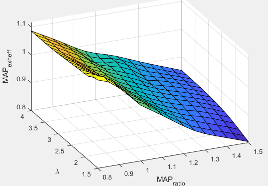#### Dependencies

To enable this parameter, for Torque model, select `Torque Structure`.

The intake manifold gas temperature exhaust temperature efficiency multiplier lookup table, ƒMATexheff, is a function of the engine speed and intake manifold gas temperature relative to optimal temperature, MATexheff = ƒMATexheff(ΔMAT,N), where:

• MATexheff is intake manifold gas temperature exhaust temperature efficiency multiplier, dimensionless.

• ΔMAT is intake manifold gas temperature relative to optimal temperature, in K.

• N is engine speed, in rpm.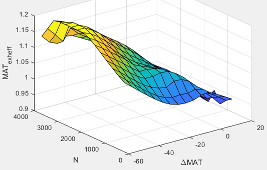#### Dependencies

To enable this parameter, for Torque model, select `Torque Structure`.

The intake manifold gas oxygen exhaust temperature efficiency multiplier lookup table, ƒO2Pexheff, is a function of the engine speed and intake manifold gas oxygen percent relative to optimal, O2Pexheff = ƒO2Pexheff(ΔO2P,N), where:

• O2Pexheff is intake manifold gas oxygen exhaust temperature efficiency multiplier, dimensionless.

• ΔO2P is intake gas oxygen percent relative to optimal, in percent.

• N is engine speed, in rpm.#### Dependencies

To enable this parameter, for Torque model, select `Torque Structure`.

The fuel rail pressure efficiency exhaust temperature multiplier lookup table, ƒFUELPexheff, is a function of the engine speed and fuel rail pressure relative to optimal breakpoints, FUELPexheff = ƒFUELPexheff(ΔFUELP,N), where:

• FUELPexheff is fuel rail pressure exhaust temperature efficiency multiplier, dimensionless.

• ΔFUELP is fuel rail pressure relative to optimal, in MPa.

• N is engine speed, in rpm.#### Dependencies

To enable this parameter, for Torque model, select `Torque Structure`.

Post-injection cylinder wall heat loss transfer coefficient, in W/K.

#### Dependencies

To enable this parameter, for Torque model, select `Torque Structure`.

 Heywood, John B. Internal Combustion Engine Fundamentals. New York: McGraw-Hill, 1988.